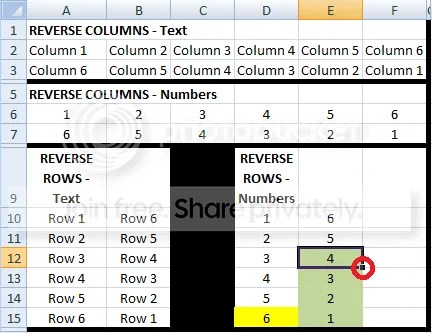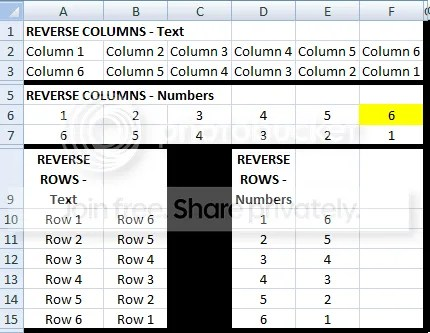Excel Worksheet Tips: Reversing Rows OR Columns of Data

There are times when you realise your list of data is in the wrong order; for example in rows 1 through 4 you have D then C then B then A. You realise you need the list to be reversed, with the order being A then B then C then D. Unfortunately, there is no formula or option that allows you to do this easily. However, it can be done!

I have searched on the internet for a while and I haven’t been able to find anything that is relatively simple. I then thought about it and came up with this solution.

REVERSING ROWS OF DATA

STEP 1 – Set your reference cell

The reference cell is the last cell of data in the list. Let’s look at an example.

Let’s first focus on the REVERSE ROWS – Numbers section found in columns D and E from rows 9 to 15. You can see how cell D15 is highlighted. This is the reference cell. This is the end of the original list; it is the value which you want to put at the START of your new list. This cell becomes the anchor for the three separate formulae that will be used to create the reverse.

If there are a large number of values in your list, you may not be able to see the last cell in the list. If this is the case you can use the keyboard combination: Ctrl + Down Arrow to get to the bottom of the list. Note: If you do not have a continuous list,( i.e. a list that has blank cells in it) then pressing this combination once will only take you to the location of the first blank. To ensure that you have captured all your data, continue pressing Ctrl + Down until you are sure you have reached the end of your list.

STEP 2 – CELL 1 of the reversed listDo you remember how you selected the reference cell in Step 1? This now becomes the first cell in your new list. So to start the new list in column E, the formula in E10 becomes:

= D15

Remember this cell, as it will become an important factor in the next two formulae.

STEP 3 – CELL 2 of the reverse listOnce you have set the first cell, you can then set the second cell. In this example, it is D14 and this needs to be located in E11. You could go into E11 and manually type this cell reference into the formula, but this could lead to mistakes. By using the reference cell, it will ensure that values in your list and not missed.

To do this, you need to use the OFFSET() function. Looking at the description of the OFFSET function in excel help:
“Returns a reference to a range that is a specified number of rows and columns from a cell or range of cells. The reference that is returned can be a single cell or a range of cells. You can specify the number of rows and the number of columns to be returned.”

The syntax of the formula is:

OFFSET(reference, rows, cols, [height], [width])

This is a more complicated version of the OFFSET function, but in this case, the formula simplifies to:

OFFSET(reference, rows, cols)

As we want to return the row BEFORE the reference cell, you need to move in a NEGATIVE direction. Therefore, the formula in cell E11 becomes:

OFFSET(D15,-1,0)

This will then copy the value of of cell D14 into E11.

Now, to complete the rest of the list, you could go through and change the formula to say -2, -3, -4, and so on, but this could also cause errors and is time consuming. Luckily, this step can also be automated.

STEP 4 – CELL 3 to n of the reverse listThe final formula is for the rest of the rows in the list. As I mentioned in STEP 3, you could go through and increase the number of steps you take each time, however this could lead to errors. To avoid this, another function is introduced, the COUNT() function.  Looking at the description of the COUNT function in excel help:

“The COUNT function counts the number of cells that contain numbers, and counts numbers within the list of arguments. Use the COUNT function to get the number of entries in a number field that is in a range or array of numbers.”

Why is count important? Currently we are trying to shift D13 (the third last value in the original list) to E12 (the third value in the reversed list). We have currently shifted a total of 2 numbers to their new positions. By using this fact, we can easily select the third value.

To do this we need to COUNT the number of cells that we have already shifted into the new list. First, let’s look at the formula to shift the second cell in the list:

CELL 2 FORMULA
OFFSET(D15,-1,0)

This simple formula has the COUNT formula added to become:

=OFFSET(\$D\$15,-COUNT(\$E\$10:E11),0)

Here is the explanation:

The reference cell is still the same (D15), however instead of entering -2, the COUNT function is used. Starting from the first cell of the reversed list (E10) it the counts the number of cells have already been reversed (currently only two; which are E10 and E11). By placing the negative sign in front of the COUNT function, the OFFSET will then move up two rows. This then shifts D13 into it’s final location E12.

You may have noticed the dollar (\$) sign in the formula. This locks the reference, so when you grab the formula and pull it down (see below) the reference cell and the start location of the reversed list are locked and are not lost.This is critical, as when you pull this final formula down [=OFFSET(\$D\$15,-COUNT(\$E\$10:E11),0)] to cover the rest of the list, if the row reference is not locked, the formula will not work as desired.

The reverse is now complete! The list is now in the opposite order!

REVERSING COLUMNS OF DATA

The next question is – what about COLUMNS of data? As the formula for reversing the list order have now been defined, a very simple modification can be made to allow columns to be reversed. Let’s look at the syntax of the OFFSET function again:

OFFSET(reference, rows, cols)

Now, setting the reference cell is again done by selecting the final cell that contains your data. (In this case, it can be found by pressing Ctrl + Right). To use the formula as defined in STEP 3 above for columns, the offset and 0 need to be alternated. For example, see the column list of data in cells A6 to F6.Cell F6 is now the reference cell and the formula in A7 becomes: = F6. To switch the second value (moving E6 to B7) the formula becomes:

=OFFSET(F6,0,-1)

You can see how the 0 and -1 values have switched to correspond to shifting COLUMNS instead of ROWS. Similarly the code for list values 3 to n becomes:

=OFFSET(\$F\$6,0,-COUNT(\$A\$7:B7))

As the reference cell is F6, and the start of the new list is A7. The count of reversed cells begins from A7 and moves across the columns, rather than down in rows.

With one simple switch you can now reverse columns!

REVERSING ROWS OR COLUMNS OF TEXT DATA

This is all fine until you want to reverse cells that contain TEXT rather than numbers. The formulae for the first two cells are identical, however when trying to use the COUNT function, it will cause an error. This is because the COUNT function cannot handle text. To avoid this, swap the COUNT function for the COUNTA function. This function counts all NON-BLANK cells rather than those with just a number. In fact, you could put COUNTA into the formulae used for the reverse of numbers and it would work exactly the same.

Hopefully these steps are clear and allow you to understand how I created a quick (but not so simple) way of reversing a list of numbers, whether they are in rows or columns. I wanted the formula to be the same so that there weren’t multiple formula to remember. I cannot remember exactly how I discovered this, I think I was searching online to find this and after putting together a variety of different pieces of advice on the internet, I came up with this solution.

If you have any questions or comments, let me know!

### Come check out the directory for the rest of my excel tips!

Submeg

IT

Posted in IT and
###### Posted by:submeg

Musician, Writer and Inspirer. I discuss the projects I am working on, the services I provide and my general thoughts.